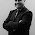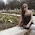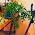MATH 1280 Introduction to Statistics – Midterm Exam – part 4

Painted by Max Ernst

Midterm Part 4 – questions 8 - 9, include its answer (total questions are 16)

Eighty adults with gum disease were asked the number of times per day they flossed. The (incomplete) results are shown below:

 # per day Freq. Rel. Freq Cum. Rel. Freq 0 15 .1875 1 21 .2625 2 18 .2250 3 .7875 4 9 .9000 5 8 1.000

One of Max Erst paintings

Fill in the blanks in the table and answer the next two question:
8) The count of people who flossed three times per day is:
Select one:
a. 11
b. 18
c. 12
d. 9 …>Correct
e. can not be determined
Answer: 80 – (15+21+18+9+8) = 9 people

Another Max Ernst painting

9) The cumulative relative frequency of those who flossed 0 to 2 times per day is:
Select one:
a. .2250
b. .7875
c. .6750 …>Correct
d. .1875
e. 0.000
answer: 0.1875 + 0.2625 +0.2250 = 0.6750

# To be continued to part 5

1.This comment has been removed by the author.

2.Interesting. My hygienist told me more than once per day is overkill. Are there really people who do it 5 times a day?

Love the Ernst paintings!

1.Thanks to visit:
MATH 1280 Introduction to Statistics – Midterm Exam – part 4
@
Midterm Part 4 – questions 8 - 9, include its answer (total questions are 16)

2.MATH 1280 Introduction to Statistics – Midterm Exam – part 4
@
9) The cumulative relative frequency of those who flossed 0 to 2 times per day is:

Select one:
a. .2250
b. .7875
c. .6750 …>Correct
d. .1875
e. 0.000
answer: 0.1875 + 0.2625 +0.2250 = 0.6750

# To be continued to part 5

3.I love statistics too. Thanks...

1.Thanks to visit:
MATH 1280 Introduction to Statistics – Midterm Exam – part 4
@
Eighty adults with gum disease were asked the number of times per day they flossed. The (incomplete) results are shown below:

2.MATH 1280 Introduction to Statistics – Midterm Exam – part 4

4.Me encantan esas pinturas y las matemáticas tambien.

1.Thanks to visit:
MATH 1280 Introduction to Statistics – Midterm Exam – part 4
@
Fill in the blanks in the table and answer the next two question:
8) The count of people who flossed three times per day is:
Select one:
a. 11
b. 18
c. 12
d. 9 …>Correct
e. can not be determined
Answer: 80 – (15+21+18+9+8) = 9 people

5.Posts Full of Art and humanism.
A very interesting try.
And thank you very much for your comment in my Blog.

T ime full of
a rts for a
n ice trip with
z eal
a nd knowledge

Warmest greetings from Volos.

1.Thanks to visit:
MATH 1280 Introduction to Statistics – Midterm Exam – part 4
@
9) The cumulative relative frequency of those who flossed 0 to 2 times per day is:

Select one:

a. .2250

b. .7875

c. .6750 …>Correct

d. .1875

e. 0.000

answer: 0.1875 + 0.2625 +0.2250 = 0.6750

2.MATH 1280 Introduction to Statistics – Midterm Exam – part 4

6.Thosr paintings are great ☺

1.Thanks to visit:
MATH 1280 Introduction to Statistics – Midterm Exam – part 4
@
Midterm Part 4 – questions 8 - 9, include its answer (total questions are 16)

7.Interesting subject. I ll wait for the next post.
Xoxo

marisasclosetblog.com

1.Thanks to visit:
MATH 1280 Introduction to Statistics – Midterm Exam – part 4
@
Eighty adults with gum disease were asked the number of times per day they flossed. The (incomplete) results are shown below:

8.Ernst's artwork is amazing. The math is tricky!

1.Thanks to visit:
MATH 1280 Introduction to Statistics – Midterm Exam – part 4
@
Fill in the blanks in the table and answer the next two question:
8) The count of people who flossed three times per day is:
Select one:
a. 11
b. 18
c. 12
d. 9 …>Correct
e. can not be determined
Answer: 80 – (15+21+18+9+8) = 9 people

2.MATH 1280 Introduction to Statistics – Midterm Exam – part 4

9.Beautiful paintings

1.Thanks to visit:
MATH 1280 Introduction to Statistics – Midterm Exam – part 4
@
9) The cumulative relative frequency of those who flossed 0 to 2 times per day is:
Select one:
a. .2250
b. .7875
c. .6750 …>Correct
d. .1875
e. 0.000
answer: 0.1875 + 0.2625 +0.2250 = 0.6750

2.MATH 1280 Introduction to Statistics – Midterm Exam – part 4

10.Good luck with the math. Hope it goes well. I am grateful that I have a job that doesn't require a lot of math work.

1.Thanks to visit:
MATH 1280 Introduction to Statistics – Midterm Exam – part 4
@
Midterm Part 4 – questions 8 - 9, include its answer (total questions are 16)

Eighty adults with gum disease were asked the number of times per day they flossed. The (incomplete) results are shown below:

2.MATH 1280 Introduction to Statistics – Midterm Exam – part 4

11.Very original paintings.

Best regards

1.Thanks to visit:
MATH 1280 Introduction to Statistics – Midterm Exam – part 4
@
Fill in the blanks in the table and answer the next two question:
8) The count of people who flossed three times per day is:
Select one:
a. 11
b. 18
c. 12
d. 9 …>Correct
e. can not be determined
Answer: 80 – (15+21+18+9+8) = 9 people